Mobile QR Code1. (Department of Information and Communication Engineering, Sungkyunkwan University. They are now with Samsung Electronics)
2. (Department of Information and Communication Engineering, Sungkyunkwan University)

Minimum energy point, subthreshold circuit, ultra-low voltage, ultra-low power

## I. INTRODUCTION

Energy-efficient computation is recognized as one of the key issues in designing battery-powered electronic devices. Especially, ultra-low-power devices such as human body implantable medical electronic devices (1) require operations with extremely low energy to let them stay active for a noticeably long period. Voltage scaling is one of the most effective ways to achieving the goal because the switching energy of digital CMOS circuits can be quadratically reduced by scaling the supply voltage (2). In an extreme case, near- or sub-threshold CMOS (3-5) allows the circuit to operate at a supply voltage similar to or lower than the threshold voltage of MOS transistors, resulting in a dramatic reduction of the switching energy. But, unfortunately, severe degradation of speed in the subthreshold CMOS circuits lets the leakage energy be increased since the time required to perform a given task increases proportionally, during which the leakage current continues to flow steadily. These opposite trends of the switching energy and the leakage energy for supply voltage scaling have brought about a need for identifying a minimum energy point (MEP) (4) for achieving a maximum possible power reduction, which is defined as the supply voltage at which the total energy of a digital CMOS circuit can be minimized. This MEP is not fixed, and can vary depending on the workload given to the circuit and the temperature at which the circuit is operating.

Fig. 1. Total energy, active energy, leakage energy, and propagation delay curves to show the minimum energy point (0.25 V) for a 50-stage inverter chain.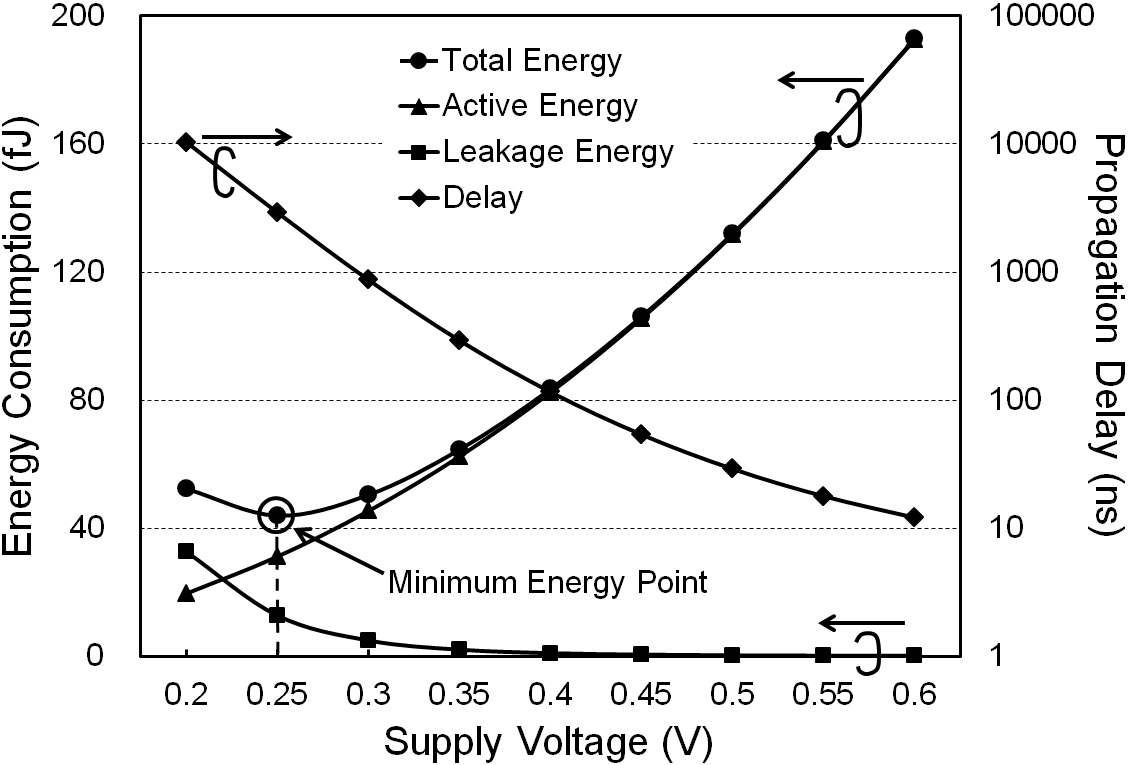A minimum energy tracking loop (6) using an embedded DC-DC converter (7) was proposed, which has several limitations due to the fact that the total energy consumed by the load system is measured by intentionally changing the supply voltage for the load system. First of all, the amount of energy measured by the loop inevitably has an error proportional to the amount of supply voltage change. For example, if the load system is consuming much energy so that the change of the supply voltage during the sensing period becomes large, the error of the measured energy becomes correspondingly very large, resulting in a deviation from true MEP. Secondly, the energy of the load system cannot be measured accurately due to the limitations of circuit components. For example, if the load system consumes very little energy so that the supply voltage change during sensing becomes negligible (1 mV or less), the error due to the offset of the comparator detecting the supply voltage change becomes dominant, preventing an exact measurement of the total energy consumed. As the third limitation, the tracking voltage step of the conventional loop is limited to 50 mV or higher. Setting up the voltage step too small might lead the conventional loop to settling at a non-minimum energy point due to errors (6). Nowadays, however, the supply voltages for smartphones and smart pads need to be fine controlled, say by a step of 10 mV or less, for finding an operating energy level as close to the true minimum energy as possible. A voltage difference of 50 mV can cause a large error on measured minimum energy in accordance with applications or characteristics of CMOS processes.

In this paper, an improved minimum energy tracking (MET) hardware is proposed to address the problems of the conventional loop explained above. Section II summarizes the energy of digital CMOS circuits. Section III describes the architecture and operation principle of the proposed MET hardware. Section IV, the measurement results are presented to verify the performance. Finally, we present the conclusion in Section V.

## II. ENERGY OF DIGITAL CMOS CIRCUITS

The total energy consumed by a digital CMOS circuit can be divided into active energy and leakage energy. Fig. 1 shows the active energy, the leakage energy, the total energy, and the propagation delay curves of a 50-stage inverter chain, where the minimum energy point occurs at a supply voltage of around 0.25 V. The active portion of the total energy of a digital CMOS circuit can be given as

##### (1)
$$E_{A C T I V E}=C_{E F F} \times V_{D D}^{2}=Q_{A C T I V E} \times V_{D D}$$

where $C_{EFF}$ is the average effective switched capacitance, ${Q}_{ACTIVE}$ is the active charge consumed by the circuit, and ${V}_{DD}$ is the supply voltage. As seen in (1), the active energy decreases quadratically with decreasing ${V}_{DD}$, which can also be recognized by the active energy curve in Fig. 1. The leakage portion of the total energy can be written as

##### (2)
$$E_{\text {LEAK}}=I_{\text {LEAK,AVG}} \times T_{D} \times V_{\text {DD}}=Q_{\text {LEAK}} \times V_{\text {DD}}$$

where ${I}_{LEAK,AVG}$ is the average leakage current, ${T}_{D}$ is the operation period, and${Q}_{LEAK}$ is the total leakage charge consumed by the circuit for the given operation period. As can be seen by the leakage energy curve in Fig. 1, the leakage energy is very small as compared to the active energy in the super-threshold region, but sharply increases in the subthreshold region. This is because the time to complete a given task increases exponentially as supply voltage is reduced to the subthreshold region, during which the leakage energy is steadily consumed. Combining (1) and (2), the total energy can be written as

##### (3)
\begin{equation*} E_{TOT}=E_{\textit{ACTIVE}}+E_{LEAK}=Q_{\textit{ACTIVE}}\times V_{DD}+Q_{LEAK}\times V_{DD}\\ =(Q_{\textit{ACTIVE}}+Q_{LEAK})\cdot V_{DD}=Q_{TOT}\times V_{DD} \end{equation*}

where ${Q}_{TOT}$ is total charge consumed by the circuit. As seen in (3), ${E}_{TOT}$ can be calculated by the product of ${Q}_{TOT}$ and ${V}_{DD}$, which implies that, for finding the minimum energy of a digital CMOS circuit, ${Q}_{TOT}$ needs to be measured as accurately as possible at a given supply voltage. As mentioned previously, the opposite trends of the active and leakage energies of a digital CMOS circuit for ${V}_{DD}$ change gives rise to a minimum energy supply voltage, which usually appears in the subthreshold region. A feedback loop for accurately finding this voltage is described in the next section.

Fig. 2. Overall block diagram of the proposed minimum energy tracking loop.## III. PROPOSED MINIMUM ENERGY TRACKING HARDWARE

Fig. 2 shows overall block diagram of the proposed MET hardware, which is comprised of an energy sensing unit, a minimum energy finder, a linear voltage regulator, a buck converter, a DAC, an oscillator, and a load system. The energy sensing unit calculates a quantity (${E}_{PROP}$) that is proportional to the total energy consumed by the load system at a specific supply voltage. The minimum energy finder finds the minimum energy point of the load system by monitoring the values of ${E}_{PROP}$ at various supply voltages, and outputs the result as a 6-bit digital value (${DV}_{REF}$). The DAC converts the digital ${V}_{REF}$ (${DV}_{REF}$) into an analog ${V}_{REF}$ (${AV}_{REF}$) to be used as the reference voltage for the buck converter or the linear voltage regulator. During the whole period for energy sensing, in which the energy sensing unit measures the amounts of energy consumed at various supply voltages, the load system is powered by the linear voltage regulator, whose output stays constant in each step in the energy sensing period (see Fig. 4). During this period, the buck converter is powered off. After the loop finds the minimum energy point, the buck converter takes turn to power the load system with the supply voltage, at which the load system operates with the minimum energy. The oscillator mimics the critical path of the load system, and automatically scales the clock frequency as the supply voltage for the load system changes.

### A. Energy Sensing Unit

Fig. 3 shows the block diagram of the energy sensing unit, which consists of a ring oscillator, a frequency-to-digital converter (FDC), and a multiplier. A bias current (${I}_{OSC}$) scaled from the regulator output current (${I}_{REG}$) is fed into the ring oscillator, which is used to charge and discharge internal nodes of the oscillator. Thus, the operating frequency ${f}_{OSC}$ can be written as (8)

##### (4)
\begin{equation} f_{OSC}=\frac{I_{OSC}}{N_{OSC}C_{OUT}V_{DD\_ EXT}}=\frac{I_{REG}}{mN_{OSC}C_{OUT}V_{DD\_ EXT}} \end{equation}

where ${N}_{OSC}$ is the number of stages, ${C}_{OUT}$ is the output capacitance of each oscillator stage, and ${V}_{DD\_ EXT}$ is the supply voltage. Constant $m$ is the scaling factor for obtaining ${I}_{OSC}$ from ${I}_{REG}$. As seen in (4), ${f}_{OSC}$ is proportional to ${I}_{OSC}$ and thus to ${I}_{REG}$. During the energy sensing period, ${I}_{REG}$ continues to change according to the given workload, and thus, ${f}_{OSC}$ also continues to change proportionally. Since the load system is powered by the linear regulator during this period, the total charge ( ${Q}_{TOT}$) consumed by the load system can be obtained by integrating ${I}_{REG}$ during whole energy sensing period ${T}_{ES}$, and can be written as

##### (5)
\begin{equation} Q_{TOT}=\int _{0}^{T_{ES}}I_{REG}dt=mN_{OSC}C_{OUT}V_{DD\_ EXT}\times \int _{0}^{T_{ES}}f_{OSC}dt \end{equation}

Integrating both sides by defining average quantities gives

##### (6)
\begin{equation} Q_{TOT}=I_{REG,AVG}\times T_{ES} \\ =mN_{OSC}C_{OUT}V_{DD\_ EXT}\times f_{OSC,AVG}\times T_{ES} \end{equation}

where ${I}_{REG,AVG}$ is the average current and ${f}_{OSC,AVG}$ is the average frequency during ${T}_{ES}$. Since the product of ${f}_{OSC,AVG}$ and ${T}_{ES}$ in (6) is equal to the number of toggles (${N}_{TOG}$) at the output of the oscillator during ${T}_{ES}$, the total charge consumed by the load system during the energy sensing period can be written as

##### (7)
\begin{equation} Q_{TOT}=mN_{OSC}C_{OUT}V_{DD\_ EXT}\times N_{TOG} \end{equation}

This result indicates that the total charge consumed by the load system during the energy sensing period can be found by counting the toggle number of the ring oscillator. To exploit this result, the frequency-to-digital converter in the energy sensing unit converts the toggle count of the ring oscillator into a digital value (${N}_{TOG}$), as seen in Fig. 3. ${N}_{TOG}$ is set to have 10 bits to cover a wide range of workload. Note that, as seen by (3) and (7), multiplying ${N}_{TOG}$ (proportional to ${Q}_{TOT}$) to ${DV}_{REF}$ (a digital equivalent to ${V}_{DD\_ INT}$) will result in a value proportional to the total energy consumed by the load system. More specifically, ${E}_{PROP}$ (=${N}_{TOG}$·${DV}_{REF}$) calculated by the multiplier in the energy sensing unit is a 16-bit digital data proportional to energy consumed by the load system at a given load supply voltage.

As explained in Section I, the minimum energy tracking loop in (6) has the problem that the measured energy can have an error due to the load supply voltage change during the energy sensing period. In contrast, the proposed energy sensing unit supplies a stable voltage and current to the load system through a voltage regulator during each step in the energy sensing period, resulting in a total elimination of such error. Another problem of the energy sensing circuitry in (6) comes from the error due to the offset of the comparator detecting the supply voltage change of the load system when the amount of change is very small. That is, if the load system consumes very little energy, the supply voltage change will become negligibly small, resulting in an increased error of the conventional energy sensing circuitry due to the offset of the comparator. The proposed energy sensing unit instead employs a current-starved ring oscillator whose operating frequency is accurately proportional to the amount of current consumed by the load system, letting the error of the proposed energy sensing unit kept small. Therefore, the proposed minimum energy tracking loop can measure energy consumption more accurately than the conventional scheme.

### B. Minimum Energy Tracking Algorithm

Fig. 4. Flowchart of the proposed minimum energy tracking algorithm.After ${E}_{PROP}$, a quantity that is proportional to the total energy consumed by the load system, is obtained by the energy sensing unit, the minimum energy finder in Fig. 2 identifies the minimum energy point using the tracking algorithm described in this section. The flowchart of the proposed minimum energy point tracking algorithm is shown in Fig. 4, which has two tracking loops: a coarse tracking loop and a fine tracking loop. As the first step, the coarse tracking loop calculates ${E}_{PROP}$ with an initial ${DV}_{REF}$, which is stored in register ${E}_{PROP,REG}$. After ${DV}_{REF}$ is decreased by coarse voltage step ${V}_{STEP,COARSE}$(50 mV), the loop recalculates and compares ${E}_{PROP}$ with the value stored in ${E}_{PROP,REG}$. If current ${E}_{PROP}$ is smaller (larger) than ${E}_{PROP,REG}$, the loop keeps on decreasing (increasing) ${DV}_{REF}$ by the same step and updating ${E}_{PROP,REG}$ until new ${E}_{PROP}$ becomes higher than the value stored in ${E}_{PROP,REG}$. If that happens, the coarse tracking loop ends and the fine tracking loop starts where the direction for the tracking is put in reverse. This loop updates ${E}_{PROP,REG}$ by changing ${DV}_{REF}$ by fine voltage step ${V}_{STEP,FINE}$ (10 mV) as far as current ${E}_{PROP}$ is lower than the previous ${E}_{PROP}$. When the fine tracking loop first finds new ${E}_{PROP}$ larger than the previous ${E}_{PROP}$, the loop ends after returning ${DV}_{REF}$ by one fine voltage step back to get to the minimum energy point.

The energy minimization point tracking algorithm proposed in the paper lets the load system approach to the vicinity of the minimum energy point by the coarse tracking loop, and finds the exact minimum energy point by the fine tracking loop. Since the coarse voltage step is as much as 50 mV, the proposed tracking loop has a merit of quickly approaching to the vicinity of the minimum energy point. Since the fine voltage step is as small as 10 mV, the loop has another merit of accurately finding the true minimum energy point. The tracking accuracy is good due to the fine tracking loop, and the overall tracking speed is also good due to the coarse tracking loop.

## IV. MEASUREMENT AND DISCUSSION

To evaluate the performance of the proposed scheme, the conventional loop in (6) and the proposed loop whose load system is an inverter chain array are designed in a 130 nm CMOS process. A 100-stage inverter chain is used as a unit load system. To compare the accuracy of tracking loops, each loop was set to be the worst-case condition at which the amount of the minimum energy measured by each tracking loop gives the maximum error. Fig. 5(a) and (b) compare the normalized worst-case minimum energy measured by each tracking loop versus light and heavy workloads, respectively. In these figures, TRUE indicates the true minimum energy curve as a reference to compare the accuracy of the minimum energy obtained by each loop. As Fig. 5(a) and (b) indicate, the amount of minimum energy measured by the proposed loop is very close to and almost the same as the true minimum energy levels at light and heavy workloads, respectively, as compared to the conventional loop. Fig. 5(c) and (d) compares the percentage error between the $true$ minimum energy and the minimum energy measured by each loop. The error of the minimum energy measured by the conventional loop is up to 3.1% for light workloads (3~9 times of unit workload) and up to 38.9% for heavy workloads (30~90 times of unit workload), respectively. Meanwhile, the error of the minimum energy measured by the proposed loop is just up to 0.16% for light workloads (3~9 times of unit workload) and 0.24% for heavy workloads (30~90 times of unit workload), respectively, resulting up to 95.0% and 99.4% accuracy improvement as compare to the conventional loop. The evaluation result indicates that the proposed loop has an ability to identify the true minimum energy level very accurately.

To assess practical applicability, the proposed MET loop with a variable word-length multiplier (9) as the load system was fabricated. The variable word-length multiplier has two operation modes: 16x16 and 32x32 modes. In the 16x16 mode, the lower 16-bit multiplicand and multiplier are used, and unused inputs and partial product blocks are forced to be zero. In the 32x32 mode, all inputs and partial product blocks are used. This multiplier employs modified Booth algorithm and Wallace tree structure and is able to operate down to 250 mV supply voltage. Fig. 6 shows the die photo of the test chip containing the MET hardware and the load system, occupying 1.43x0.64 mm$^{2}$. The MET loop circuitry except the multiplier occupies 0.66x0.64 mm$^{2}$.

Fig. 5. Minimum energies at (a) light workload, (b) heavy workload, percentage errors of measured minimum energy at (c) light workload, (d) heavy workload.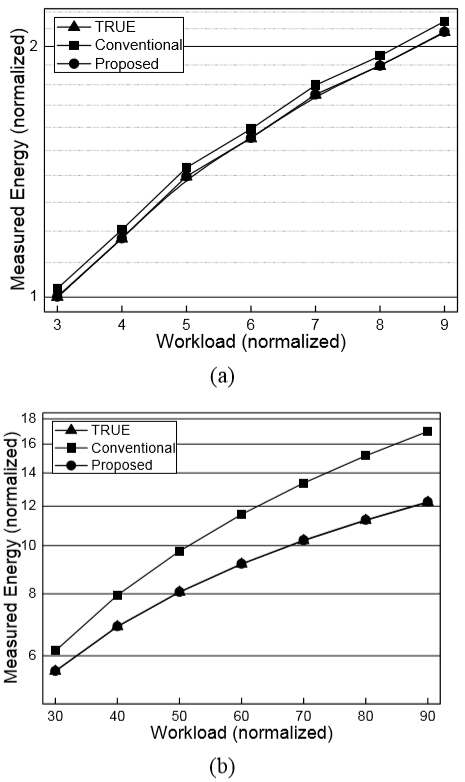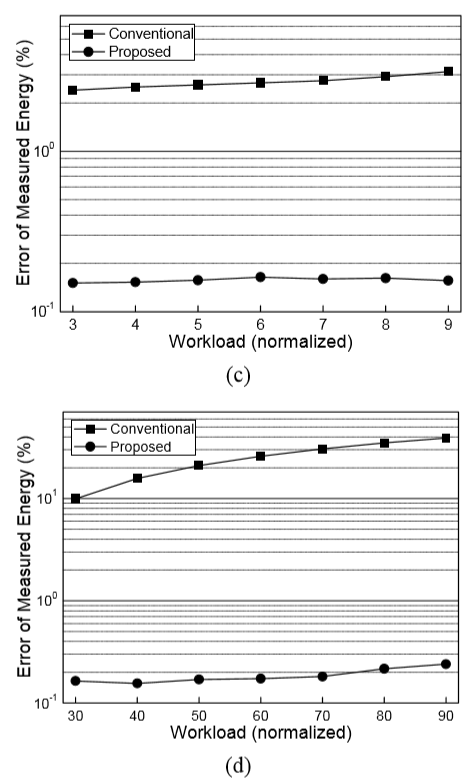Fig. 7. Measured waveforms of the proposed MET loop with different workloads (a) 32x32 mode, (b) 16x16 mode.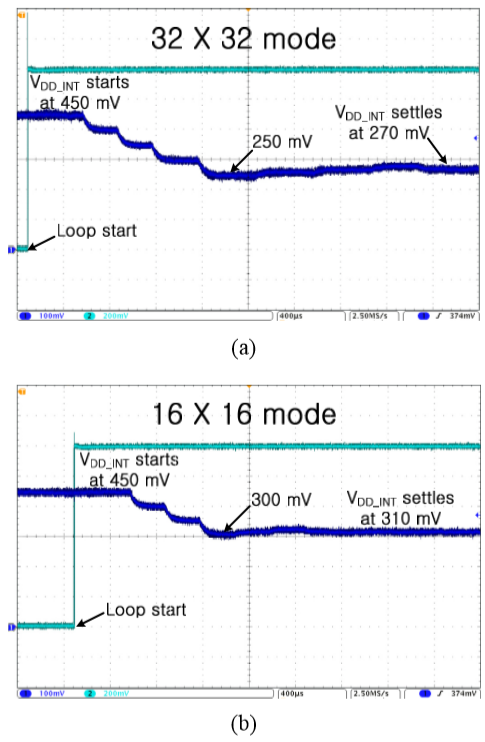Fig. 7 shows measured waveforms of the proposed MET hardware with the load system operating at each operation mode: Fig. 7(a) for the 32x32 operation mode and Fig. 7(b) for the 16x16 operation mode. From Fig. 7(a), it can be seen that the coarse tracking loop begins with ${V}_{DD\_ INT}$ at 450 mV, and searches the minimum energy point by lowering ${V}_{DD\_ INT}$ (= ${DV}_{REF}$) by a coarse voltage step of 50 mV. Recognizing that the new ${E}_{PROP}$ at 250 mV becomes higher than the previous ${E}_{PROP}$, the coarse tracking loop is switched to the fine tracking loop that starts by increasing ${V}_{DD\_ INT}$ by a fine voltage step of 10 mV. As soon as the fine tracking loop finds the new ${E}_{PROP}$ at 280 mV to become larger than the previous ${E}_{PROP}$, the proposed loop recognizes that the minimum energy point of the multiplier occurs at 270 mV. As Fig. 7(b) shows, the proposed tracking loop finds by a similar procedure that the minimum energy point of the multiplier operating at the 16x16 mode occurs at 310 mV.

Fig. 8. Measured energy versus supply voltage with change in workload.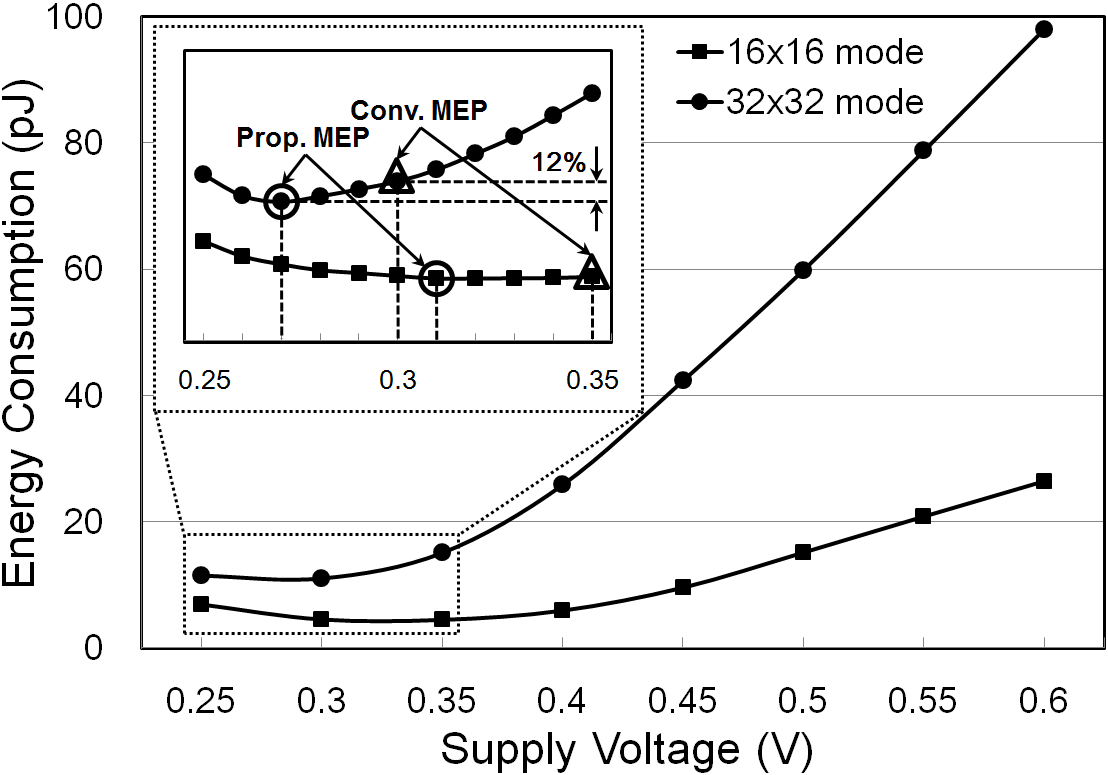Fig. 9. Measured energy of 16x16 multiplier mode at various temperatures.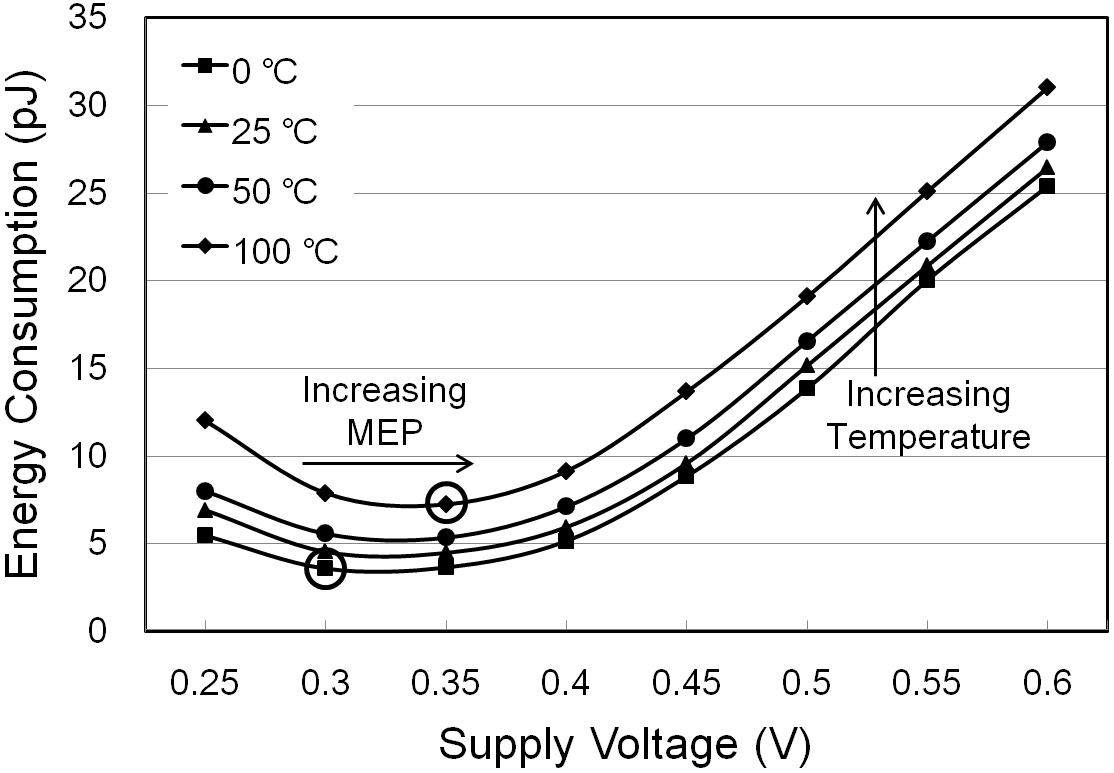Fig. 8 depicts the measured energy of the variable word-length multiplier with changing the supply voltage. The figure indicates that the more the workload is given to the load system, the lower the minimum energy point becomes. This is expected since the ratio of the active energy to the leakage energy at a same supply voltage becomes higher as workload increases. The points marked with rings and triangles on the curves are MEPs measured with tracking steps of 10 mV and 50 mV, respectively. As shown in this figure, the minimum energy consumption of the multiplier measured with 10-mV step was reduced by up to 12% as compare to the minimum energy consumption of the multiplier measured only with 50-mV step. Thus, the proposed MET hardware that tracks the MEP by a step of 10 mV can find more accurate minimum energy operating voltage as compare to conventional loop that tracks the MEP only by 50 mV step. The MEP measured in 10 mV of each multiplier mode appears respectively at 310 mV and 270 mV. Fig. 9shows that the MEP on the 16x16 multiplier mode increases as temperature is increased from 0 $^{\circ}$C to 100 $^{\circ}$C. This result is also expected since an increase of temperature induces an increase of the leakage energy.

## V. CONCLUSIONS

In this paper, a minimum energy tracking (MET) loop is proposed, which can allow a load system to consume the minimum energy for a given workload and temperature. The proposed minimum energy tracking loop can track the minimum energy point more accurately than the conventional design by adopting an accurate energy sensing method and a hierarchical tracking scheme. Experimental result in 130-nm CMOS process indicated that the proposed loop can find the true minimum energy point far more accurately than the conventional loop can do, resulting in about 12% more energy saving when a variable word-length multiplier is used as the load system. According to our evaluation, it is expected that, if the proposed minimum energy tracking loop is applied to a full-chip level design like a microprocessor, the energy saving will be getting substantially larger.

### ACKNOWLEDGMENTS

This work was partly supported by the National Research Foundation of Korea (NRF) grant funded by the Korean Government (MSIT) (No. 2019R1A2C1011155) and by the Institute of Information & Communications Technology Planning & Evaluation (IITP) grant funded by the Korean Government (MSIT) (No.2019-0-00421, AI Graduate School Support Program (Sungkyunkwan University)).

### REFERENCES

1
Potocny M., 2018, Self Vth-compensating CMOS on-chip rectifier for inductively powered implantable medical devices, IEEE International Symposium on Design and Diagnostics of Electronic Circuits and Systems, pp. 158-1612
Yang K., 2017, Hardware designs for security in ultra-low-power IoT systems: an overview and survey, IEEE Micro, Vol. 37, No. 6, pp. 72-893
Manolov E. D., 2018, Design of CMOS analog circuits in subthreshold region of operation, IEEE Int. Scientific Conference Electronics, pp. 1-44
Mohammad S., 2017, A low-power temperature-compensated CMOS peaking current reference in subthreshold region, in IEEE International Symposium on Circuits and Systems, pp. 1-45
Park J.-H., Feb 2017, Conditional-Boosting Flip-Flop for Near-Threshold Voltage Application, IEEE Trans. on VLSI Systems, Vol. 25, No. 2, pp. 779-7826
Ramadass Y., Jan 2008, Minimum Energy Tracking Loop with Embedded DC-DC Converter Enabling Ultra-Low-Voltage Operation Down to 250 mV, IEEE J. Solid-State Circuits, Vol. 43, No. 1, pp. 256-2657
Suh J.-D., March 2019, A fast response PWM buck converter with active ramp tracking control in a load transient period, IEEE Trans. on CAS-II, Vol. 66, No. 3, pp. 467-4718
Hwang Y.-S., Feb 2009, Low-sensitivity low-bounce high-linearity current-controlled oscillator, IEEE Trans. on Ultrasonic, Ferroelectric, and Frequency Control, Vol. 56, No. 2, pp. 254-2629
Takayanagi T., 1996, 350 MHz time-multiplexed 8-port SRAM and word size variable multiplier for multimedia DSP, in IEEE ISSCC Dig. Tech. Papers, pp. 150-151## Author

received the B.S. and the M.S. degrees in electrical engineering from Korea Aerospace University, Goyang, Korea, in 2003 and 2005, respectively, and the Ph.D. degrees in electrical engineering from Sungkyunkwan University, Seoul, Korea, in 2013.

In 2013, he joined System LSI business, Samsung Electronics Co. Ltd., Suwon, Korea, where he is currently a staff engineer at Design Technology team and involved in the design of custom circuits for mobile AP and CIS products.

He is conducting research on high-speed, low-power mobile SOC and Sensor design methodology, and his recent research interests include AI processor design.

received the B.S. degrees from the Department of Electronic and Electrical Engineering from Sungkyunkwan University, Suwon, Korea, in 2015, where he is currently pursuing the Ph.D. degree with the Department of Electrical and Computer Engineering.

His current research interests include the design of analog integrated circuits, computing in memory, and hardware-friendly neural networks.

received the B.S. degrees in Department of Electronic and Radio Engineering from KyungHee University, Youngin, South Korea, in 2013, and the M.S. and the Ph.D. degree in Department of Electrical and Computer Engineering from Sungkyunkwan University, Suwon, South Korea, in 2020.

In 2019, he joined System LSI Business, Samsung Electronics, Hwasung, South Korea, where he is involved in the design of LDOs and DC-DC converters.

His current research interests include Power Management IC design.

received the B.S degree in school of information and communication engineering from Korea Aerospace University, Goyang, Korea, in 2004, and M.S and Ph.D degrees in electrical engineering from Sungkyunkwan University, Suwon, Korea, in 2007 and 2013, respectively.

From 2013 to 2016, he joined System LSI business, Samsung Electronics, Co, Ltd., Suwon, Korea.

In 2016, he joined Foundry business, Samsung Electronics, Co, Ltd., Suwon, Korea, where he is currently a staff engineer at IP Development team and involved in the design of analog IP for mobile AP and foundry products.

He is currently conducting research on small-area low-power temperature sensor, analog security detector, and voltage regulator design.

(SM’94, M’00) received the B.S. degree in electronics engineering from Yonsei University, Seoul, Korea, in 1990, and the M.S. and the Ph.D. degrees in electrical engineering from Korea Advanced Institute of Science and Technology (KAIST), Taejon, Korea, in 1992 and 1996, respectively.

From 1996 to 1999 he was with LG Semicon (currently Hynix Semiconductor), Seoul, Korea, as a senior design engineer, where he was working on the design of high-density and high-bandwidth DRAMs.

In 2000, he joined the faculty of Korea Aerospace University, Goyang, Korea, as an assistant professor at the School of Electronics Telecommunication and Computer Engineering.

In 2005, he moved to Sungkyunkwan University, Suwon, Korea, where he is currently a professor at the College of Information and Communication Engineering.

His research interests include high-performance processor and memory architecture/circuit designs, high-speed transceiver design, neuromorphic integrated circuit design, and IC designs for low-power and high-speed application.